edge-connectivity

Kriesell's Conjecture ★★

Author(s): Kriesell

Conjecture   Letbe a graph and let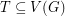such that for any pairthere are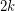edge-disjoint paths fromtoin. Thencontainsedge-disjoint trees, each of which contains.

Partitioning edge-connectivity ★★

Author(s): DeVos

Question   Letbe an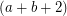-edge-connected graph. Does there exist a partitionof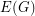so thatis-edge-connected andis-edge-connected?

Keywords: edge-coloring; edge-connectivity

(2 + epsilon)-flow conjecture ★★★

Author(s): Goddyn; Seymour

Conjecture   For everythere exists an integerso that every-edge-connected graph has a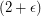-flow.

Keywords: edge-connectivity; flow